##### Circuit Analysis For DummiesYou can extend an inverting amplifier to more than one input to form a summer, or summing amplifier. An inverting amplifier takes an input signal and turns it upside down at the op amp output.

Here is an inverting op amp with two inputs. The two inputs connected at Node A (called a summing point) are connected to an inverting terminal.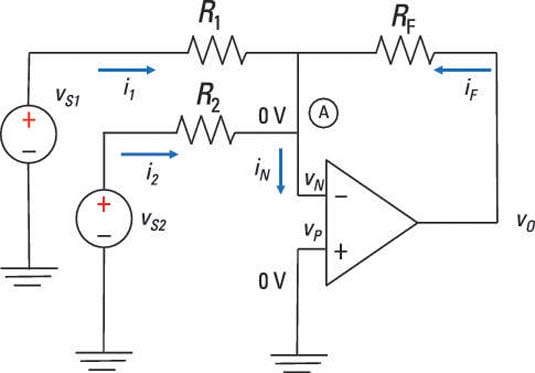Because the noninverting input is grounded, Node A is also connected as a virtual ground. Applying the KCL equation at Node A, you wind up with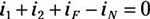Replace the input currents in the KCL equation with node voltages and Ohm’s law (i = v/R):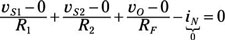Because iN = 0 for an ideal op amp, you can solve for the output voltage in terms of the input source voltages: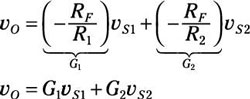The output voltage is a weighted sum of the two input voltages. The ratios of the feedback resistance to the input resistances determine the gains, G1 and G2, for this op amp configuration.

To form a summing amplifier (or inverting summer), you need to set the input resistors equal with the following constraint: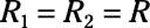Applying this constraint gives you the output voltage: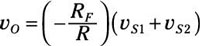This shows that the output is proportional to the sum of the two inputs. You can easily extend the summer to more than two inputs.

Plug in the following values for the sample circuit to test this mumbo jumbo: vS1 = 0.7 volts, v2 = 0.3 volts, R1 = 7 kΩ, R2 = 3 kΩ, and RF = 21 kΩ. Then calculate the output voltage vo: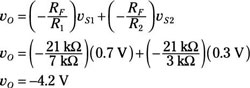The signals are bigger — mission accomplished. If signals are changing in time, the summer adds these signals instantly with no problem.